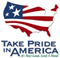Open-File Report 2007–1194

# Documentation of the U.S. Geological Survey Oceanographic Time-Series Measurement Database

## Conversion Factors

International System of Units to Inch/Pound

Multiply By To obtain
Length
centimeter (cm) 0.3937 inch (in.)
meter (m) 3.281 foot (ft)
kilometer (km) 0.6214 mile (mi)
Volume
cubic centimeter (cm3) 0.06102 cubic inch (in3)
Velocity
centimeter per second (cm/s) 0.03281 foot per second (ft/s)
meter per second (m/s) 3.281 foot per second (ft/s)
Mass
kilogram (kg) 2.205 pound avoirdupois (lb)
Pressure
decibar (dbar) 10,000 pascal (Pa)
millibar (mb) 100 pascal (Pa)
Density
kilogram per cubic meter (kg/m3) 0.06242 pound per cubic foot (lb/ft3)

Temperature in degrees Fahrenheit (°F) may be converted to degrees Celsius (°C) as °C = (°F x 32) / 1.8.

Supplemental Information

Concentrations of chemical constituents in water are given either in milligrams per liter (mg/L) or micrograms per liter (µg/L)

Frequency is given in hertz (Hz), equivalent to cycles per second.

Conductivity is given in siemens per meter (S/m).

Light-emitting diode (LED) wavelength is given in nanometers (nm; 1/1,000,000,000 meter).

Nitrate concentration is given in micromoles per liter (µmol/L)U.S. Department of the Interior | U.S. Geological Survey
URL: http://pubsdata.usgs.gov/pubs/of/2007/1194/ofr2007-1194-convert.html
Page Contact Information: GS Pubs Web Contact